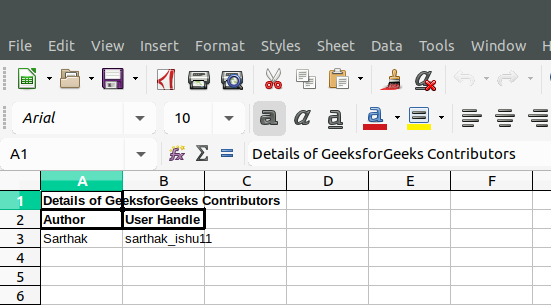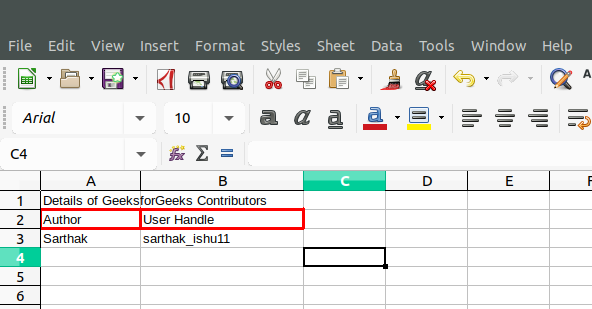Related Articles
PHP | Spreadsheet_Excel_Writer | Close() Function
• Difficulty Level : Medium
• Last Updated : 21 Dec, 2018

The close() function is an inbuilt function in PHP | Spreadsheet_Excel_Writer which is used to finalize the spreadsheet after which all the operations are done. This method should always be the last one to be called on every workbook.

Syntax:

`mixed Workbook::close()`

Parameters: This function does not accept any parameter.

Return Value: This function returns TRUE on success and PEAR_ERROR on failure.

Example 1:

 `addFormat(); ` ` `  `// Set Bold Format  ` `\$format_bold``->setBold(); ` ` `  `// Add Worksheet to Sprerdsheet ` `\$worksheet` `=& ``\$workbook``->addWorksheet(); ` ` `  `\$format_bold``->setBorder(2); ` ` `  `// Write to Worksheet ` `\$worksheet``->write(0, 0, "Details of GeeksforGeeks  ` `                   ``Contributors", ``\$format_bold``); ` `\$worksheet``->write(1, 0, ``"Author"``, ``\$format_bold``); ` `\$worksheet``->write(1, 1, ``"User Handle"``, ``\$format_bold``); ` `\$worksheet``->write(2, 0, ``"Sarthak"``); ` `\$worksheet``->write(2, 1, ``"sarthak_ishu11"``); ` ` `  `// Send .xlsx file to header ` `\$workbook``->send(``'test.xls'``); ` ` `  `// Close Workbook Object ` `\$workbook``->close(); ` `?> `

Output:Example 2:

 `addFormat(); ` ` `  `// Add Worksheet to Sprerdsheet ` `\$worksheet` `=& ``\$workbook``->addWorksheet(); ` ` `  `// Set and Add Border Width ` `\$format_border``->setBorder(2); ` ` `  `// Set and Add Color to Border ` `\$format_border``->setBorderColor(``'red'``); ` ` `  `// Write to Worksheet ` `\$worksheet``->write(0, 0, "Details of GeeksforGeeks  ` `                     ``Contributors", ``\$format_bold``); ` `\$worksheet``->write(1, 0, ``"Author"``, ``\$format_border``); ` `\$worksheet``->write(1, 1, ``"User Handle"``, ``\$format_border``); ` `\$worksheet``->write(2, 0, ``"Sarthak"``); ` `\$worksheet``->write(2, 1, ``"sarthak_ishu11"``); ` ` `  `// Send .xlsx file to header ` `\$workbook``->send(``'test.xls'``); ` ` `  `// Close Workbook Object ` `\$workbook``->close(); ` `?> `

Output:My Personal Notes arrow_drop_up
Recommended Articles
Page :